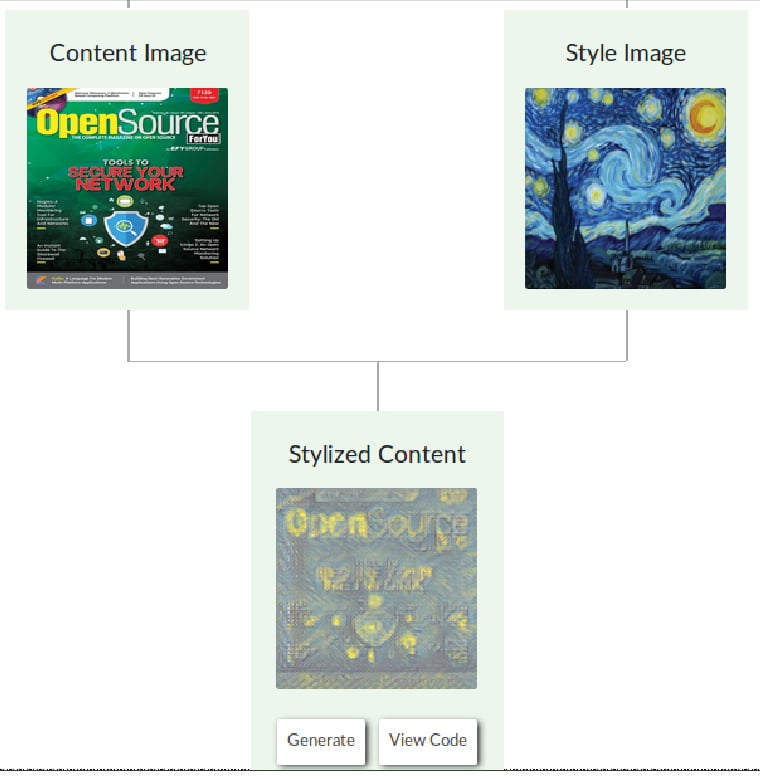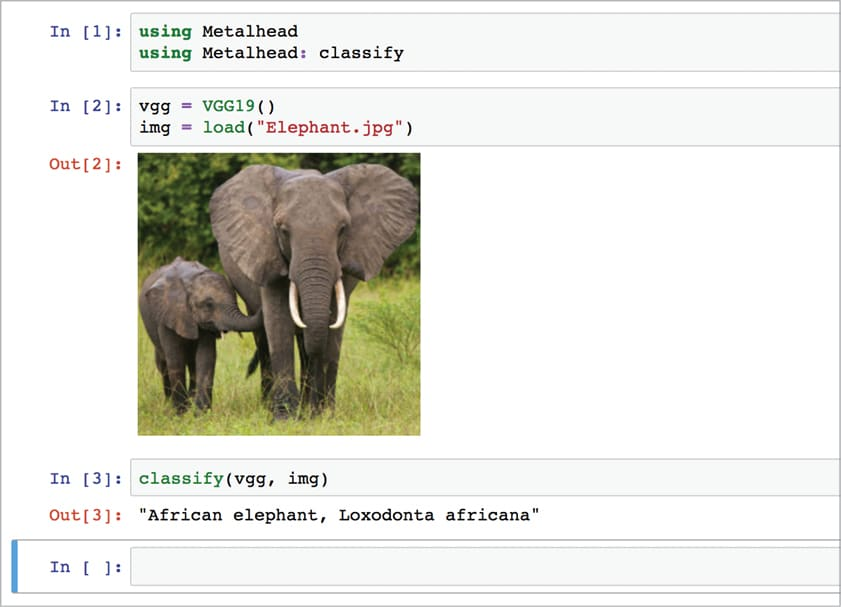# The Best Machine Learning Libraries in Julia

0
1699Advertisement

Machine learning (ML) is ubiquitous these days. This article introduces useful machine learning libraries in Julia, the developer base for which is growing fast. With the inherent advantages that Julia has in processing mathematical expressions, implementation of ML is very effective. This article takes you through popular libraries in Julia such as Flux, Knet, MLBase.jl and TensorFlow.jl.

Machine learning is everywhere, and developers are trying to adopt it across all domains. The adoption of ML has changed the fundamental thought process behind building solutions. ML has given a new dimension to the process of building software solutions by changing it from a step-by-step approach into a learning-based approach. The inherent advantage here is the ability of the program to learn the intrinsic, hidden patterns in the data and to react to novel scenarios.

There are ML libraries available in many programming languages. C++, Python and R are popularly used in the ML arena. This article explores the popular machine learning libraries of Julia, a programming language that has combined the simplicity of Python with the speed of C++. Hence, by using Julia, you can get the best of both worlds – simplicity combined with speed. The Julia developer base has been steadily increasing over recent months. It has got applications in a wide spectrum of domains.

One of the prominent features of Julia is its ability to handle mathematical expressions with elegance and speed. If you have some exposure to ML, you might be aware that its core concepts are completely mathematical. Hence, by adopting Julia for machine learning, we get the inherent advantage of effective expression handling to optimise the machine implementations.

Advertisement

Julia provides a wide variety of options for building ML solutions by offering various libraries. Not just for ML, Julia in general is very rich in terms of the libraries and packages it offers. A complete list is provided in the Julia Observer page (https://juliaobserver.com/). Even the list of libraries that Julia offers to build ML solutions alone is very long, as shown in Figure 1.Figure 1: Julia machine learning options

This article provides a brief introduction to the following libraries in Julia:

• Flux
• Knet
• MLBase.jl
• TensorFlow.jl
• ScikitLearn.jl

Flux

Flux is a popular choice for building machine learning solutions in Julia. This can be inferred from the Julia Observer Web page, which has higher ratings for Flux. The following are the advantages of using Flux:

• It is a very lightweight option.
• The entire Flux code is written in Julia. This is unlike libraries in some other languages for which the interface is in one language and the core components are in another such as C++.
• Another big advantage of Flux is that it comes with many built-in tools. It is fully loaded with features that are required for building a ML solution.
• Flux can be used along with other Julia components such as data frames and differential equation solvers.

You can start using Flux straight away without complex installation processes. You just need to use Pkg.add, as shown below:

`Pkg.add(“Flux”)`

As the official documentation says, Julia is simply executable math, just like Python is the executable pseudo code. Hence the conversion of mathematical expressions into working code is elegant in Julia.

To start with, there are some executable Flux experiments listed in the https://fluxml.ai/experiments/ page. You can navigate and see the live demos to get an insight into the power of Flux. For example, a style transfer demo application with the cover page of OSFY magazine is shown in Figure 2.Figure 2: Style transfer demo with OSFY cover page

The official documentation (https://fluxml.ai/Flux.jl/stable/) lists the complete set of features provided by Flux.

The Model-Zoo has various demonstrations which can be very useful in understanding the many dimensions of Flux (https://github.com/FluxML/model-zoo/). These models are categorised into the following folders:

• Vision: Large Convolutional Neural Networks (CNNs)
• Text: Recurrent Neural Networks (RNNs)
• Games: Reinforcement learning (RL)

If you are working in the field of computer vision, you may find Metalhead an interesting option. This package provides computer vision models that run on top of the Flux library. A sample code sequence to perform image content recognition is shown in Figure 3.

Overall, Flux is evolving into a major choice for ML development in Julia.Figure 3: Flux computer vision code sequence

Knet

Knet has been built by Koc University, Turkey. It is a deep learning framework written in Julia by Dr Deniz Yuret along with other contributors (https://github.com/denizyuret/Knet.jl).

The Knet framework supports GPU operations. It has support for automatic differentiation, which is carried out using dynamic computational graphs.

Similar to Flux, you can start using Knet directly without any major installation process. You need to simply add it with Pkg.add() as shown below:

`Pkg.add(“Knet”)`

If you encounter any installation issues, follow the instructions given in the official Web page at http://denizyuret.github.io/Knet.jl/latest/install.html.

A simple example of using Knet for training and testing the LeNet model for the MNIST handwritten digit recognition data set is shown below (source: https://github.com/denizyuret/Knet.jl):

```-

using Knet

# Define convolutional layer:

struct Conv; w; b; f; end

(c::Conv)(x) = c.f.(pool(conv4(c.w, x) .+ c.b))

Conv(w1,w2,cx,cy,f=relu) = Conv(param(w1,w2,cx,cy), param0(1,1,cy,1), f)

# Define dense layer:

struct Dense; w; b; f; end

(d::Dense)(x) = d.f.(d.w * mat(x).+ d.b)

Dense(i::Int,o::Int,f=relu) = Dense(param(o,i), param0(o), f)

# Define a chain of layers:

struct Chain; layers; end

(c::Chain)(x) = (for l in c.layers; x = l(x); end; x)

# Define the LeNet model

LeNet = Chain((Conv(5,5,1,20), Conv(5,5,20,50), Dense(800,500), Dense(500,10,identity)))

# Train and test LeNet (about 30 secs on a gpu to reach 99% accuracy)

include(Knet.dir(“data”,”mnist.jl”))

dtrn, dtst = mnistdata()

train!(LeNet, dtrn)

accuracy(LeNet, dtst)```

Knet documentation has detailed instructions for handling major deep learning building blocks, some of which are listed below:

• Back propagation
• Convolutional Neural Networks
• RNN
• Reinforcement learning

If you are a researcher working in the deep learning domain, you may find the paper titled ‘Knet: Beginning deep learning with 100 lines of Julia’ by Dr Deniz Yuret interesting.

MLBase.jl

Unlike the previous two libraries, the MLBase.jl library doesn’t implement specific algorithms used in ML. But it provides a number of handy tools to assist building ML programs. The MLBase.jl may be used for the following tasks:

• Preprocessing and data manipulation
• Performance evaluation
• Cross-validation
• Model tuning

MLBase.jl depends on the StatsBase package. Detailed usage instructions are available at the official documentation page at https://mlbasejl.readthedocs.io/en/latest/index.html.

TensorFlow.jl

TensorFlow.jl is a wrapper around the most popular ML framework from Google — TensorFlow.

TensorFlow.jl can be added using Pkg.add() as shown below:

`Pkg.add(“TensorFlow”)`

A basic usage example is shown below:

```using TensorFlow

using Test

sess = TensorFlow.Session()

x = TensorFlow.constant(Float64[1,2])

y = TensorFlow.Variable(Float64[3,4])

z = TensorFlow.placeholder(Float64)

w = exp(x + z + -y)

run(sess, TensorFlow.global_variables_initializer())

res = run(sess, w, Dict(z=>Float64[1,2]))

@test res ≈ exp(-1)```

If you are a machine learning researcher, then you may find the paper titled ‘TensorFlow.jl: An Idiomatic Julia Front End for TensorFlow’ by Malmaud and White very interesting. This paper has been published in the Journal of Open Source Software.

If you have worked with TensorFlow in Python, you may find the Julia version very similar. There are some inherent advantages of the Julia interface over Python which are illustrated in detail in the official documentation at https://github.com/malmaud/TensorFlow.jl/blob/master/docs/src/why_julia.md.

ScikitLearn.jl

ScikitLearn.jl is the Julia version of the popular Scikit-learn library. As you may be aware, ScikitLearn is a very popular option for building ML solutions.

The ScikitLearn.jl is a simple-to-use ML library in Julia with lots of features (https://scikitlearnjl.readthedocs.io/en/latest/). The major features of ScikitLearn.jl are listed below:

• Support for DataFrames
• Hyper parameter tuning
• Feature unions and pipelines
• Cross-validation

The availability of a uniform interface to Julia and Python models makes using ScikitLearn.jl effective and simple.

As stated in the beginning of this article, Julia is a powerful language when it comes to handling mathematical expressions. This core advantage is reflected in all the ML libraries built using Julia. In the near future, Julia could emerge as the leading choice for implementing machine learning solutions for a majority of developers.

Advertisement
SHAREThe author is an assistant professor of computer science at, School of Engineering and Technology, Pondicherry Central University, with over 10 years of experience in both academia and industry. He has been awarded the Best Teacher Award three times in 2010, 2011 and 2013.